Practice questions available in McGraw Hill Math Grade 2 Answer Key PDF Chapter 2 Test will engage students and is a great way of informal assessment.

Question 1.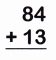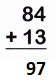By adding 84 and 13 we get the result 97.

Question 2.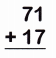By adding two numbers 71 and 17 we get 88.

Question 3.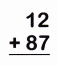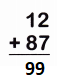By adding two numbers 12 and 87 we get 99.

Question 4.By adding two numbers 36 and 40 we get 76.

Question 5.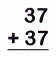By adding two numbers 37 and 37 we get 74.

Question 6.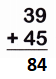By adding two numbers 39 and 45 we get 84.

Question 7.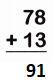By adding two numbers 78 and 13 we get 91.

Question 8.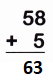By adding two numbers 58 and 5 we get 63.

Subtract. Write the difference.

Question 9.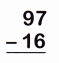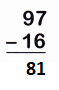By subtracting two numbers 97 and 16 we get 81.

Question 10.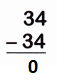By subtracting two numbers 34 and 34 we get 0.

Question 11.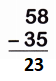By subtracting two numbers 58 and 35 we get 23.

Question 12.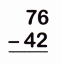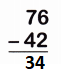By subtracting two numbers 76 and 42 we get 34.

Question 13.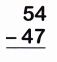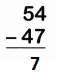By subtracting two numbers 54 and 47 we get 7.

Question 14.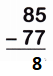By subtracting two numbers 85 and 77 we get 8.

Question 15.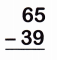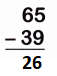By subtracting two numbers 65 and 39 we get 26.

Question 16.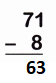By subtracting two numbers 71 and 8 we get 63.

Add or subtract. Write the sum or difference.

Question 17.
There are 58 people on a plane. Then 21 more people get on the plane. How many people are on the plane now?
___ +___ =___ people
Given,
There are 58 people on a plane.
Then 21 more people get on the plane.
58 + 21 = 79 people
There are 79 people on the plane now.

Question 18.
One box has 48 crayons. Another box has 24 crayons. How many more are in the box of 48?
___ – ___ = ___ crayons
Given,
One box has 48 crayons.
Another box has 24 crayons.
48 – 24 = 24 crayons

Write the number sentence for both steps. Add and subtract to solve. Write the answer.

Question 19.
Tara has 20 stickers. She gives 18 stickers away. Then Tara gets 12 more stickers. How many stickers does Tara have now?
____ – ____ = ____
___ + ___ = ___ stickers
Given that,
Tara has 20 stickers. She gives 18 stickers away.
20 – 18 = 2 stickers
Then Tara gets 12 more stickers.
2 + 12 = 14 stickers
Thus Tara has 14 stickers now.

Question 20.
There are 26 books on one shelf in the classroom.
There are 38 books on another shelf.
The students take 20 of the books to read.
How many books are still on the shelves?
___ + ___ = ___
____ – ___ = ___ books
There are 26 books on one shelf in the classroom.
There are 38 books on another shelf.
26 + 38 = 64 books
64 – 20 = 44 books
Thus there are 44 books on the shelves.

Solve for the unknown number. Write a number sentence. Write the answer.

Question 21.
58 +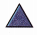= 94= ____
58 += 94= 94 – 58= 36

Question 22.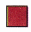– 27 = 33= ____– 27 = 33= 33 + 27= 60

Question 23.
89 –= 53= ____
89 –= 53
89 – 53 == 89 – 53= 36

Question 24.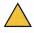+ 74 = 99= ___+ 74 = 99= 99 – 74= 25